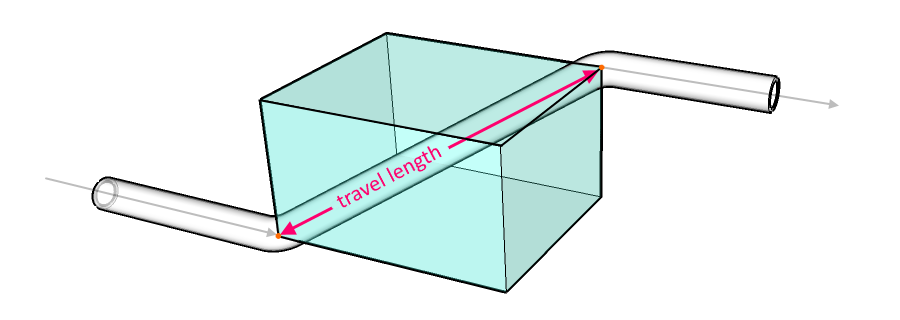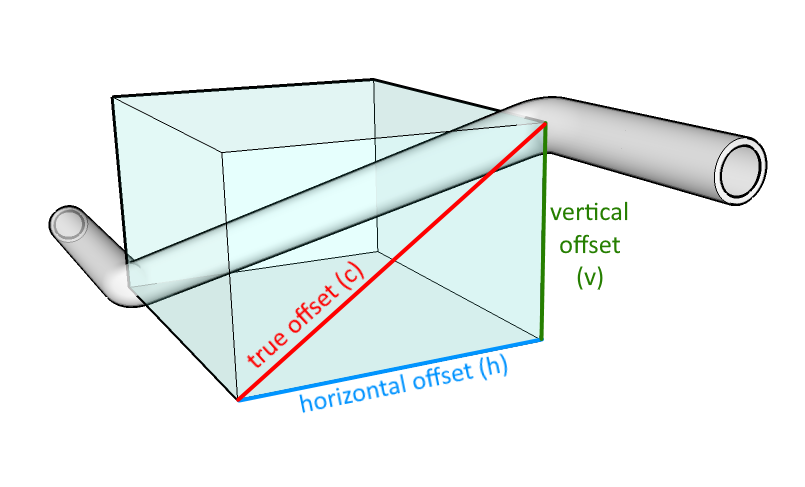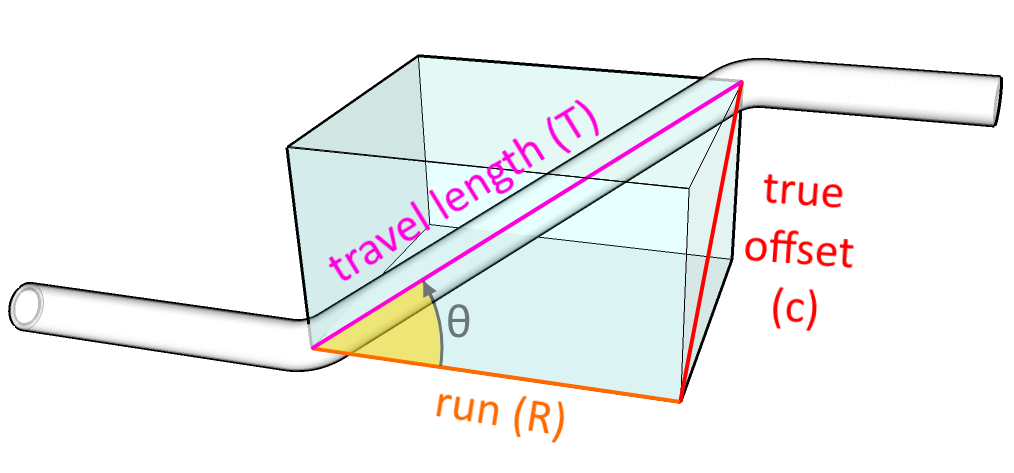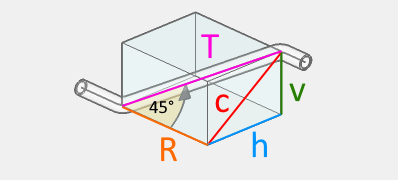# Rolling Offset Calculator

Created by Kenneth Alambra
Reviewed by Wojciech Sas, PhD candidate and Steven Wooding
Last updated: Aug 03, 2021

This rolling offset calculator, or pipe offset calculator, will help you find the travel distance needed to complete a necessary pipe offset for any typical pipe fitting bends or any bend angle you prefer.

In this calculator, you will learn:

• What rolling offset is;
• The different pipe rolling offset formulas; and
• How to calculate rolling offsets.

You will also read about the different terms we usually use when dealing with pipe offsets like true offset, travel, and run. Additionally, we will show you a pipe offset chart containing pipe offset multipliers that you can use to find the length of travel quickly and easily.

## What is a pipe offset? What is a rolling offset?

In doing some pipe works, we sometimes encounter situations wherein we have to connect two lines of pipes that are not quite aligned with each other. These two pipelines could be offset horizontally or vertically. When we see a combination of both horizontal and vertical offset, or in other perspectives, a combination of offsets in two different directions, we call it a pipe rolling offset.

To deal with pipe offsets, we can use a bent pipe fitting to change the direction of the pipeline and another bent fitting to return to the final direction of the pipeline. In this calculator, we tackle how to find the travel length, which is the length of the diagonal that connects the two points at which the pipeline changes directions, as shown in the image below:The length of travel depends on the horizontal and vertical offsets and bent pipe fitting used. We can work out the length of travel using trigonometry, some concepts behind right triangles, and the Pythagorean theorem. Before we proceed on how to calculate rolling offsets, let us first learn how to use our pipe offset calculator.

## How do I use the rolling offset calculator?

Using our pipe rolling offset calculator is effortless as you don't need to know any rolling offset formula for this. Our tool can also help you visualize what your rolling offset looks like with diagrams that change according to your selection and inputs. Here are the steps you can follow to use our calculator:

1. Enter both the horizontal and vertical offsets needed for your pipeline. Our pipe offset calculator will instantly show you the true offset of your pipeline.
2. Then, choose your preferred fitting bend, depending on which is available to you. Select Custom if you are bending your own pipe or if you got custom-angle bent pipe fittings.
3. If you picked Custom from the Fitting bend selection, our tool will display the variable Bend angle of fittings to input your desired bend angle.
4. Upon selecting or entering your fitting bend, you will then see the length of travel and the length of run your pipeline needs to follow to complete your pipe rolling offset.

In the next section of this text, we'll learn how to calculate rolling offsets ourselves to understand better how our calculator works.

## How do I calculate the pipe rolling offset?

Figuring out a rolling offset in piping is a matter of finding two hypotenuses of two right triangles inside the imaginary box a rolling offset forms. In the illustration below, we can see that we can consider the horizontal and vertical offset measurements as the legs of a right triangle, and its hypotenuse is the true offset of the pipeline:Using the Pythagorean theorem, we can determine the value of the true offset c, which is simply the diagonal of a rectangle, using the following equation:

c = √(h² + v²),

where:

• cTrue offset of the pipeline;
• hHorizontal offset of the pipeline; and
• vVertical offset of the pipeline.

Now let's take a look inside the imaginary box to see another right triangle formed by the true offset, the run, and the travel length, with the help of this illustration:In this triangle, we cannot use the Pythagorean theorem since we initially do not know the value of the run R, which is needed to obtain the length of travel T. However, since we know the bend angle of the pipe fitting we want to use, we can utilize the trigonometric function of sine to get the value of T, as with the equation below:

T = c / sin(θ),

where:

• TLength of travel of pipe that connects the two opposite corners of our imaginary box;
• cTrue offset of the pipeline; and
• θBend angle of the pipe fitting you will use.

You can then use the Pythagorean theorem to find the length of the run, using this equation:

R = √(T² - c²),

or the trigonometric function of a tangent, as shown here:

R = c / tan(θ).

Apart from these equations, it is also worth noting that in case you already know the value of run, together with the values of horizontal and vertical offsets, you can directly obtain the value of T using this equation:

T = √(R² + h² + v²).

Now that we know all the equations we might need in calculating rolling offset measurements, how about we consider an example 🙂?

## How to do a 45-degree rolling offset? Sample calculation

Let's say we want to connect two pipelines that are offset horizontally and vertically by a meter (100 cm) and half a meter (50 cm), respectively. In this situation, we plan to use 45-degree pipe elbows to complete the rolling offset. So how do we do this 45-degree rolling offset? We can start by obtaining the true offset of the pipeline using our equation:

c = √(h² + v²)

c = √(100² + 50²) = √(10000 + 2500)

c = √(12500) = 111.80 cm

Then, using our equation for solving the length of travel, we have:

T = c / sin(θ)

T = 111.80 / sin(45°)

T = 158.11 cm

We can then get how long the run would be by using either of the two equations discussed earlier. In this example, let's try using the one with the tangent function, as follows:

R = c / tan(θ)

R = 111.80 / tan(45°)

R = 111.80 cm

We now know the necessary distances to complete our pipeline's rolling offset with the calculations we made. Furthermore, notice that the run is always equal to the true offset whenever we use 45-degree elbows. For other pipe fitting angles, their values will already be different.

💡 Take note, though, that you need to adjust the travel length according to your fitting allowances to determine the necessary pipe length to cut for the perfect assembly of your pipeline.

## How do I figure rolling offset in piping fast? The use of pipe offset multipliers

Another way to determine the travel length is by using some pipe offset multiplier constants. These constants will come in handy, especially when you want to calculate travel lengths yourself, but you only have a calculator that doesn't have trigonometric functions. We have these constants in the pipe offset chart below for your reference:

Fitting bend
Multiplier
22 ½°
2.6131
45°
1.4142
60°
1.1547
90°
1.0000

To use a pipe offset multiplier, though, you first have to evaluate the true offset. Then to find the travel length, you have to multiply the constant by the true offset, as shown in the equation below:

T = m * √(h² + v²),

where m is the pipe offset multiplier.

## FAQ

### What is a rolling offset?

When we need to offset a pipeline in horizontal and vertical directions, we have a rolling offset. Imagine a pipeline that enters a corner of an imaginary box and exits the farthest opposite diagonal corner of the said imaginary box. You can complete a rolling offset by finding what is called the travel length of the pipe.

### How do I calculate rolling offset in piping?

To calculate rolling offset in piping:

1. Measure the rolling offset's horizontal and vertical offsets.
2. Determine the true offset, which is the hypotenuse of the right triangle formed by these offsets.
3. Divide the true offset by the sine of the fitting's bend angle to find the travel length.
4. Additionally, you can divide the true offset by the tangent of your fitting's bend angle to estimate the rolling offset's run.

### How do I figure a rolling 45 offset?

To figure a rolling offset using 45-degree bent fittings:

1. Determine the horizontal and vertical offsets of your pipeline.
2. Take the square root of the sum of the horizontal and vertical offsets' squares. This will be the true offset of your rolling offset.
3. Finally, multiply the true offset by 1.4142 or divide the true offset by sin(45°).

### How do I glue PVC pipe fittings?

The best way to glue PVC pipe fittings onto pipes is by using PVC solvent cement. PVC solvent cements temporarily melt the surfaces of the PVC materials you apply them on. While these surfaces of the PVC melt, you can put them into contact with each other to form a chemical bond as it dries. Make sure to twist the pipe and the fitting a bit to ensure a properly sealed connection when using PVC solvent cement.

### How do I find the travel of a pipe?

To find the travel of a pipe:

1. Measure the horizontal offset, vertical offset, and run of the rolling offset.
2. Square each of these measurements, then add them all together.
3. Take the square root of the sum to get the travel.
4. If you don't know the value of run or have a preferred bent fitting angle, repeat steps 2 and 3 with run equal to zero and divide everything by the sine of your fitting's bend angle.

### What is the travel of a 1x1-foot 45-degree rolling offset?

To find the travel length of a rolling offset with 1-foot horizontal offset and 1-foot vertical offset using a 45-degree bent fitting:

1. Square the horizontal and vertical offsets and add them together like so: 1² + 1² = 2.
2. Evaluate its square root to find the true offset: √2 = 1.41421 ft.
3. Finally, divide 1.41421 ft by the sine of 45° to get 1.41421 ft/sin (45°) = 2 ft.
Kenneth AlambraRoll or horizontal offset (h)
in
Set or vertical offset (v)
in
True offset (c)
in
Fitting bend
45°
Travel (T)
in
Run (R)
in
People also viewed…

### Car heat

The hot car calculator shows you how fast the interior of a car heats up during a summers day.

### Concrete

Concrete calculator - slab evaluate the amount of concrete and cement needed for a slab block.

### Retaining wall

Use the retaining wall calculator to estimate both the amount of materials needed and the price of your project.

### Steps to calories

Steps to calories calculator helps you to estimate the total amount to calories burned while walking.